# Rooting

If a is a real number and n is a natural number, then every solution of the equation
xⁿ = a after x in the set R, if any, is called the nth root of a.

The root is denoted (read: nth root of a). The number n is called the root pointer, the number a is the sub-root expression, and the symbol √ is the root character.

When n is an odd number,it makes sense for any a ∈ R, and when n is an even number, then the symbol only makes sense if a. 0.

The procedure by which it is determined is called rooting.

The following is true of the root:when n is an odd number;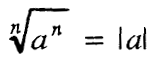when n is an even number.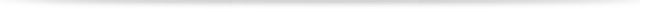## 1. EXPANDING AND SHORTENING THE ROOTS

1) If a is a positive real number and m, n and p are natural numbers, thenThis property is called root extension.

2) If the roots are given and there is a common divisor k for the numbers m and n, then:This property is called root shortening.## 2. RADICATION OF PRODUCT AND QUANTITY

1) If a and b are positive real numbers, then for every natural number n the equation holds:Note. The rule for multiplication of roots by equal root indicators is given by the law.

If two or more multiplicative roots have different root indices, they should first be reduced to roots with equal indices.

2) If a and b are positive real numbers, then for every natural number n, the equation is true: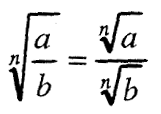Note. Pawenitygives the rule for dividing the root by equal root pointers.## 3. HOPMAL TYPE OF ROOT

### 3.1. Extract the multiplier before the root sign

If the root is given, where m> n and m = np + q, and> 0, then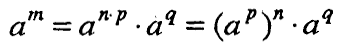In that case, ap is extracted before the sign of the root, and aq thnnuvn in the root, ie.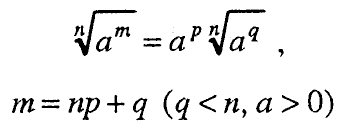### 3.2. Enter a multiplier before the root sign

The procedure for entering a multiplier under the sign of the root is the opposite of that for extracting a multiplier before the sign of the root, ie. if there is a multiplier in front of the root to enter under the root sign, it should be scaled with the root pointer first.

### 3.3. Normal root type

A root is said to be in the normal form (simplified form) when the rooted expression:
– does not contain a denominator;
– does not contain multipliers that can be extracted before the root sign;
– when the root indicator and the sub-root expression indicator do not have a common divisor.

Example.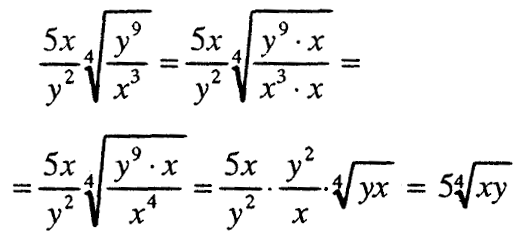If a ∈ R + and n, p ∈ N, then the equation is true:## 5. ROOT ROOTING

If a ∈ R + and n, p ∈ N, then the equation is true:## 6. DEGREE WITH INDICATOR RATIONAL NUMBER

If m and n are natural numbers and if a is a positive number, then: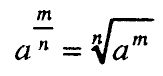M / n is called a degree with a rational indicator.## 7. IRRATIONAL EXPRESSIONS

The expressions in which it is represented and the rooting are called irrational expressions.

Irrational expressions of the type AJV, where A and B are rational expressions, are called roots. The expression A is called the coefficient of the root.

Two or more roots that are brought to normal form are called similar roots if they have equal sub-root expressions and equal root pointers.

### 7.1. Collection and removal of roots

These operations are performed with the help of transformation reduction to similar roots.

Example: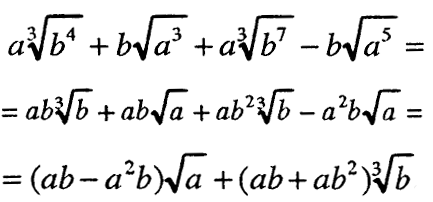### 7.2. Multiplication of irrational expressions

The multiplication of irrational expressions is done in the same way as the multiplication of rational expressions, using the rule for multiplication of roots.

Example: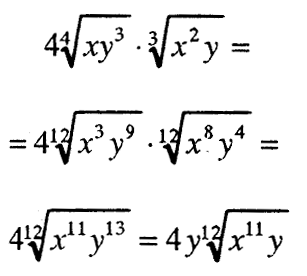### 7.3. Sharing irrational expressions

The division of irrational expressions is done as well as the division of rational expressions, using the rule of division of roots.

Example.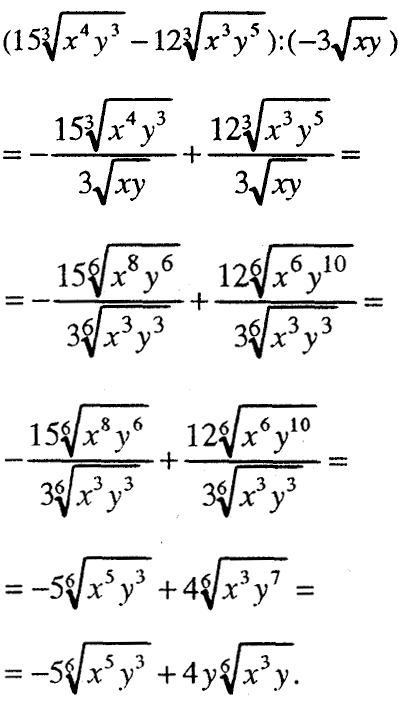### 7.4. Rationalize the fraction denominator

The transformation by which the denominator of a fraction of an irrational expression is converted into a rational expression is called rationalizing the denominator of the fraction.

1) Rationalize the denominator of the fraction of the type2) Rationalize the denominator of the fraction of the type3) Rationalize the denominator of the fraction of the type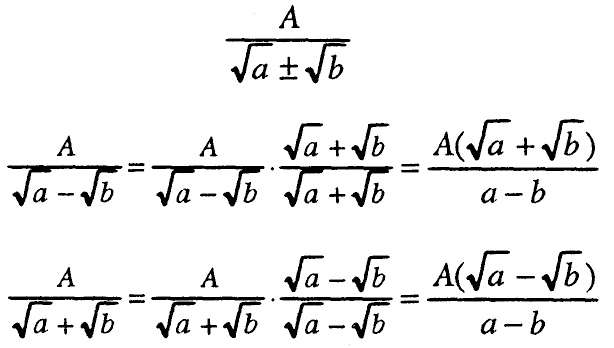###### – rooting –
Total Page Visits: 403 - Today Page Visits: 1